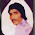Foundation, Concrete and Earthquake Engineering

### Piles in Tension in Pile Group

Generally the number of pile is determined by the axial load divided by capacity of each pile considering both end bearing and skin friction. But actual situation is there have always some reactions from lateral forces like earthquake and wind forces to piles as axial force, moment and shear force. The axial force is treated as usual. But the moment makes the differences in design and anchorage properties of both in pile and caps. In case of moment in pile cap, total reaction on each pile can be found by the expression below:Where, P=sum of reactions that are resulted from axial and moment

∑V= total vertical loads on foundation

n= number of piles in pile group
∑M=this is the sum of moments that have discussed above. The moment is not about center of gravity of cap but about center of gravity of pile group.

d= distance from the respective piles to the center of gravity of pile group.

If the p is positive there is no question of uplift and necessary anchorage. But if p is negative and piles in group are not anchored to pile caps the only a part of piles in pile group are in compression up to neutral axis. As the rest piles are not anchored to pile cap, these piles become ineffective as neither compression nor tension reactions are developed in them. The worse situation is, as some portion of piles in group is ineffective the compression reaction on the active piles is increased.

Thus it can be concluded that if piles in group are capable to withstand tension and sufficient anchorage to cap ia available the loads of each pile is calculated by the expression above.

#### 1 comment:

1.OUTSTANDING ENGINEERING INFORMATION !!